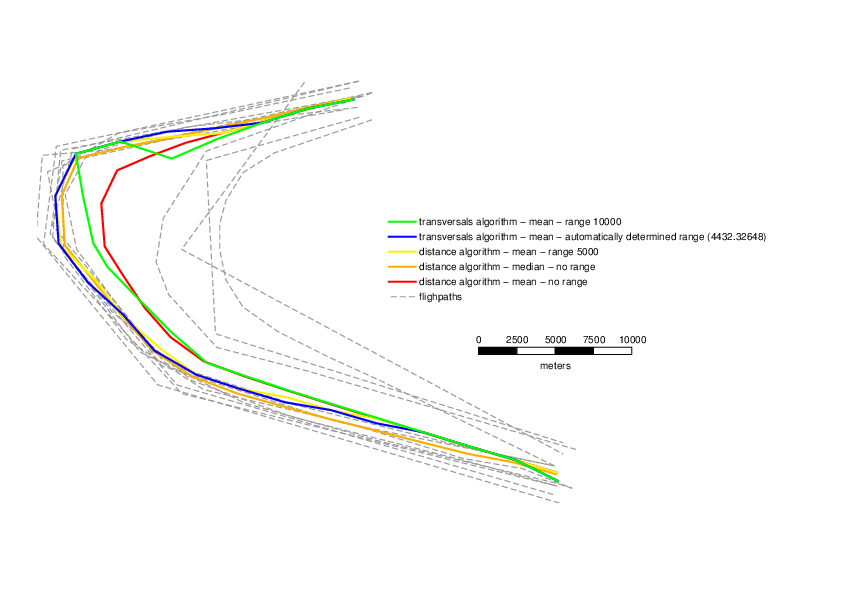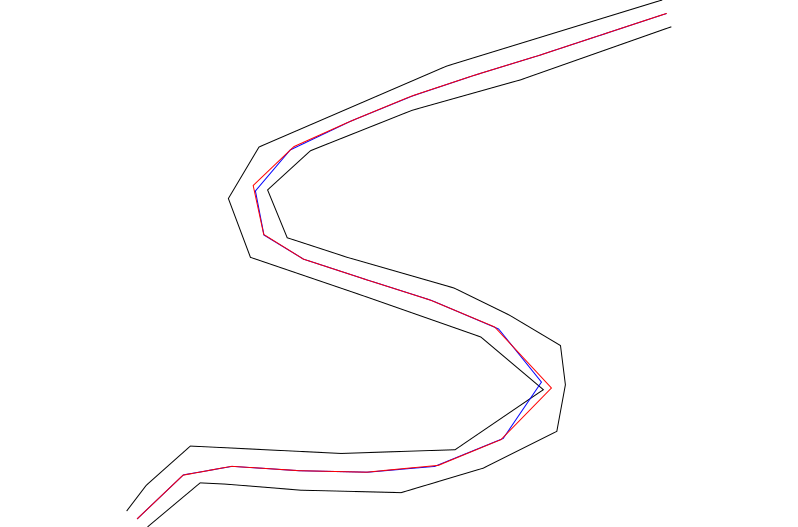Note: This addon document is for an older version of GRASS GIS that will be discontinued soon. You should upgrade your GRASS GIS installation, and read the current addon manual page.

## NAME

v.centerline - Creates a central line of a map of lines

## KEYWORDS

vector, lines central

## SYNOPSIS

v.centerline
v.centerline --help
v.centerline [-tm] input=name output=name [range=float] [refline=integer] [vertices=integer] [--overwrite] [--help] [--verbose] [--quiet] [--ui]

### Flags:

-t
Use transversal line algorithm
-m
Use approximate median instead of mean
--overwrite
Allow output files to overwrite existing files
--help
Print usage summary
--verbose
Verbose module output
--quiet
Quiet module output
--ui
Force launching GUI dialog

### Parameters:

input=name [required]
Name of input vector map
Or data source for direct OGR access
output=name [required]
Name for output vector map
range=float
Distance (in map units) of search radius
refline=integer
Category of the line to use as initial reference
vertices=integer
Number of vertices for center line
Default: 20

## DESCRIPTION

v.centerline creates a new map with a line representing an approximation of the central tendency of a series of input lines that all have similar trajectories. This can for example, be the central line of a river represented by its two sides, or a line representing the general direction of a series of flight paths, etc.

Two algorithms are proposed in the module, both based on the idea of using a reference line, creating a series of reference points along this line and then finding the coordinates of corresponding points on all the input lines. The default algorithm uses closest distance to identify corresponding points, while the second algorithm (t flag) draws perpendicular transversals at the reference points and uses the intersections of these transversals with the other lines as corresponding points.

In detail, the default algorithm goes as follows:

• choose one of the input lines as reference line
• create a series of points at regular intervals on this line
• for each of these points:
• find the closest point on each of the input lines
• get the coordinates of those points
• calculate the mean or (mathematical) median of these coordinates
• use the calculated means (or medians) as vertices of the new line

The transversals algorithm goes as follows:

• choose one of the input lines as reference line
• create a series of perpendicular (transversal) lines at regular intervals on this line
• for each of these transversals:
• find the intersection points of the transversal with all input lines
• get the coordinates of those points
• calculate the mean or (mathematical) median of these coordinates
• use the calculated means (or medians) as vertices of the new line

The user can change three parameters in the algorithms: the choice of the reference line (refline), the number of vertices to calculate (vertices) and the search range (range), i.e. for the default algorithm the maximum distance of corresponding points from the reference line and for the second algorithm the length of the transversals on each side of the reference line.

If no reference line is given the module choses the reference line by determining the mean distance of the midpoint of each line to the midpoints of all other lines. The line with the lowest mean distance is then chosen as the reference line. If no range is given, the module uses the mean of the above mean distances as the range for the transversals algorithm, and an unlimited search range for the default algorithm.

If the m flag is set and there are more than 2 lines in the input file, the module calculates the mathematical median of the x and of the y coordinates.

## NOTES

This module is more of a proof of concept showing that an approximate solution to the problem is possible with existing GRASS modules. A C-based solution would probably be much more efficient.

The median in this module is not the geometric median, but the simple mathematical median respectively of the x and the y coordinates.

The transversals algorithm is very sensitive to the range parameter. The user might want to play around with this parameter to find the best value.

Increasing the number of vertices should have a smoothing effect on the resulting line, but in the case of the transversals algorithm it can possibly lead to more instability.

## EXAMPLE

```v.centerline input=flightpaths output=center_line_mean
v.centerline -m input=flightpaths output=center_line_median
v.centerline input=flightpaths output=center_line_mean_5000 range=5000
v.centerline -t input=flightpaths output=center_line_mean_t
v.centerline -t input=flightpaths output=center_line_mean_t_8000 range=8000
```Different centerlines resulting from variations in the parameters and flags
```v.centerline input=river output=center_line
v.centerline -t input=river output=river_center_t
```Mean central line (median only makes sense if number of lines > 2) for distance (red) and transversals (blue) algorithms, the latter with automatically determined range

v.segment, v.distance

Moritz Lennert

## SOURCE CODE

Available at: v.centerline source code (history)

Latest change: Mon Jun 28 07:54:09 2021 in commit: 1cfc0af029a35a5d6c7dae5ca7204d0eb85dbc55

Note: This addon document is for an older version of GRASS GIS that will be discontinued soon. You should upgrade your GRASS GIS installation, and read the current addon manual page.

© 2003-2023 GRASS Development Team, GRASS GIS 7.8.8dev Reference Manual